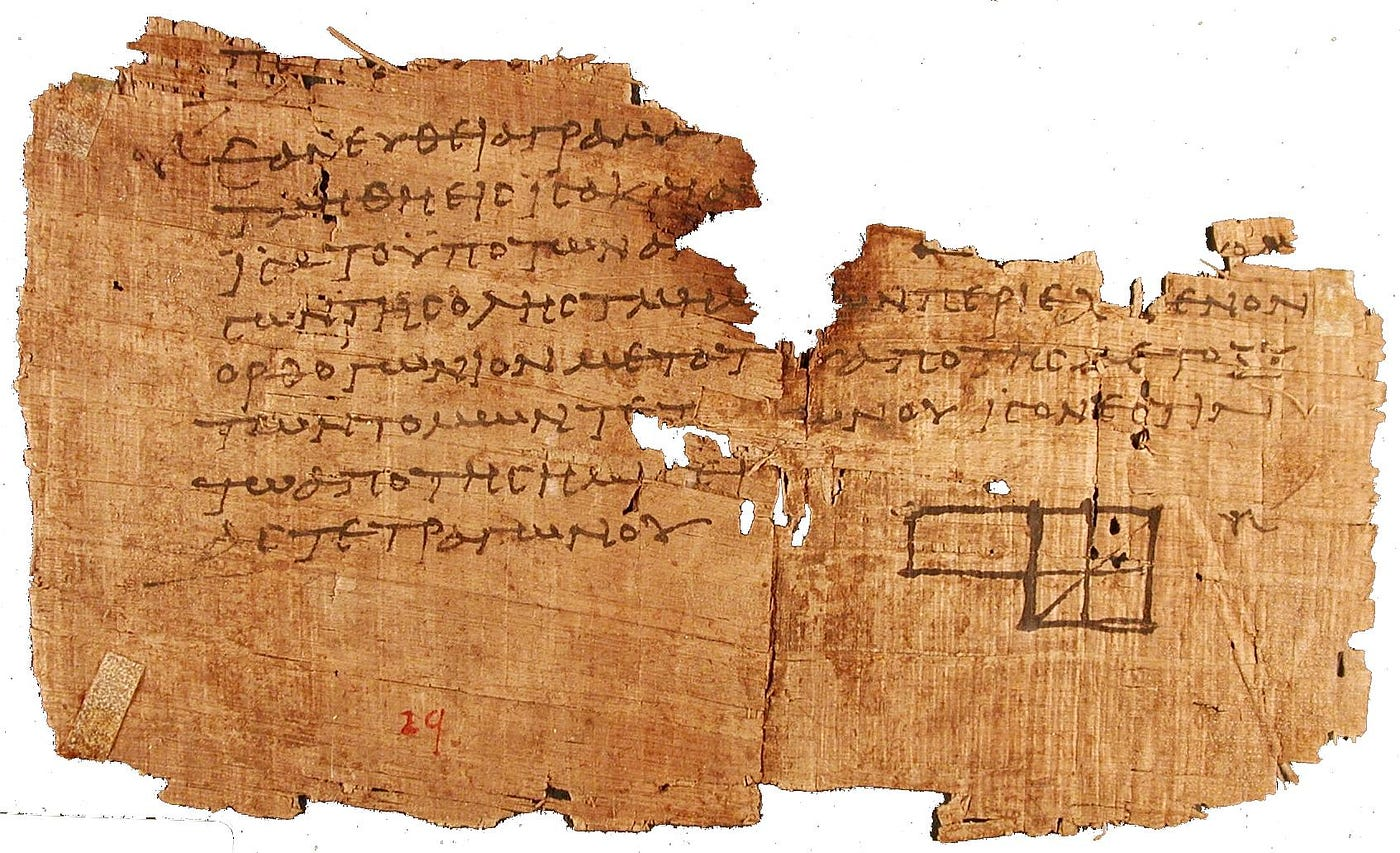One of the oldest surviving fragments of Euclid’s Elements found at Oxyrhynchus, Egypt. It was in this book that Euclid explained his algorithm to find the greatest common divisor of two numbers. This fragment was discovered in 1896 and now resides at the University of Pennsylvania.

# Ancient Algorithms: Using the Euclidean Algorithm to Find the Least Common Multiple of a Range of Numbers

Today’s post is going to break down an algorithm in JavaScript that finds the least common multiple of a range of numbers. I’ll be explaining both a basic solution that uses multiple loops and an optimized solution that makes use of both recursion and the Euclidean algorithm.

## The Challenge

First, let’s take a look at the problem we are trying to solve. The task is to write a function called findLCM that takes an array of two numbers and returns the least common multiple (LCM) of those two numbers. The LCM should be a positive integer that can be evenly divided by both of the starting numbers, as well as by all sequential numbers in the range between them. The starting array will contain two numbers that will not necessarily be in numerical order. For example, if given [5, 1], find the LCM of both 1 and 5 that is evenly divisible by all numbers between 1 and 5.

When complete, the findLCM function should return the following results for these arrays:

• [5, 1] => 60
• [8, 7] => 56
• [4, 8] => 840

## The Basic Solution

The first step in solving the problem is to make sure that the array is sorted from the greatest to lowest number. The easiest way to do this is to use the sort method on the initial arr that takes a function with parameters a and b. This function returns b — a, which sorts the array in the order we want.

Next, a new array called fullArr is declared and a for loop pushes all of the numbers from the greatest to lowest number into this array. At the end of this loop, the fullArr will contain all of the numbers within the range that we need to check against our LCM.

Three new variables are created called result, which will hold the result of each check and ultimately the final result we will be returning. The loop variable counts the iterations of the upcoming while loop. The index variable holds the index of the array that needs to be checked next.

The final portion of the solution involves a while loop that runs while the index has not reached the length of the fullArr. This will go through each number in the range to check for the LCM. Within this loop, the first number of the array is multiplied by the loop and the second number. The for loop then iterates from index 2 of the array until one less than the length of the fullArr. If the result does not divide evenly with the number at the current index then the for loop breaks. If it is even, then it checks for the next number in the array until it is not even or the final answer is found. The loop variable increases by one each time the while loop goes through. After the loop is complete, the final result is returned.

While this basic solution works, it has poor performance because of the high number of loop iterations it has to go through.

## The Optimized Solution

The optimized solution for this problem begins by determining which number in the initial array is the smaller number and which number is the larger one. This is done using the Math.min and Math.max methods that takes a value and returns the min or max of that set. For an array, this is done by using (…arr). The ellipse indicates a spread of the array (all of the elements in the array will be checked). This is slightly more efficient than the short sort done in the basic solution. A result variable is also declared to again hold the current LCM and ultimately the final result.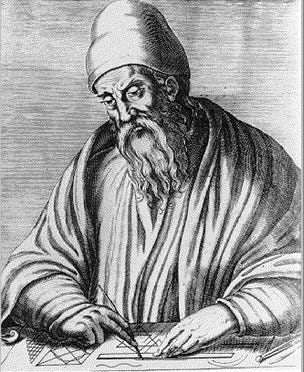A representation of the Greek mathematician Euclid, active in Alexandria, Egypt during the late 4th and early 3rd centuries B.C.E.

Next is the part you’ve been waiting for! The Euclidean algorithm. This is set up as a function called GCD — a reference to greatest common divisor, which is the largest number that divides two numbers without leaving a remainder. The Euclidean Algorithm itself is a very efficient way to find the GCD of two numbers and is named after the Ancient Greek mathematician Euclid. The GCD function uses recursion (it calls itself) whenever the second parameter does not equal zero. Using this recursion, the second parameter becomes the new first parameter and the second parameter becomes the remainder of dividing x and y. Since the recursion stops when this second parameter is zero that means it stops when the remainder of the dividing the two numbers is zero.

The final portion of the optimized solution is a for loop that runs through all of the numbers between the min and max numbers from the initial array. For the first iteration, the result is set to the LCM of the current and next number in the range. For every subsequent iteration, the result is the LCM of the previous result and the next number in the range. Finally, the LCM of the entire range is returned once the loop completes. This solution runs much more efficiently than the basic solution.

--

--

--

## More from Austin William Smith

Historian | Full Stack Developer

Love podcasts or audiobooks? Learn on the go with our new app.

## What is Collection in Java?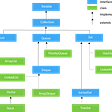## ShibarmyZilla-HMNG Collaboration## soundcloud / icem## Entering the world of IT as an outsider and complete beginner.## OS Dev as a NOOB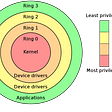## Student’s story: Ross — from beginner to a job in Madrid## Austin William Smith

Historian | Full Stack Developer

## Creating a code review checklist## Day 7 of #100daysofcode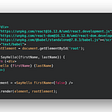## Dirty Little Web Developer Tricks and Lies## Pair Programming